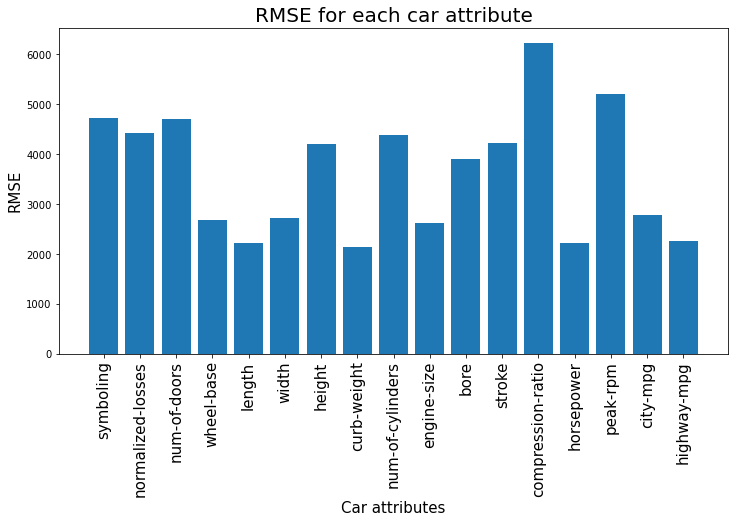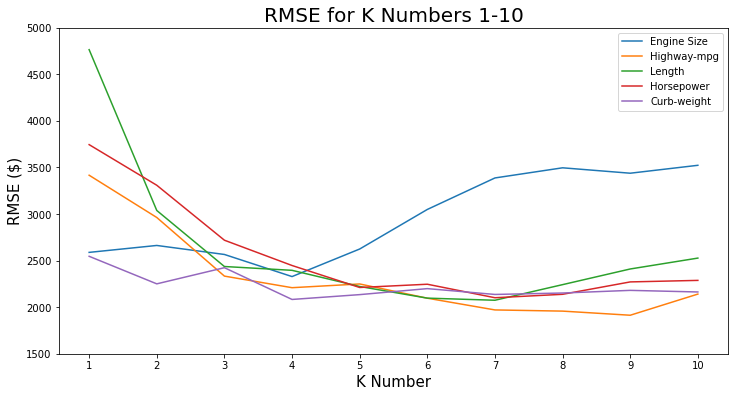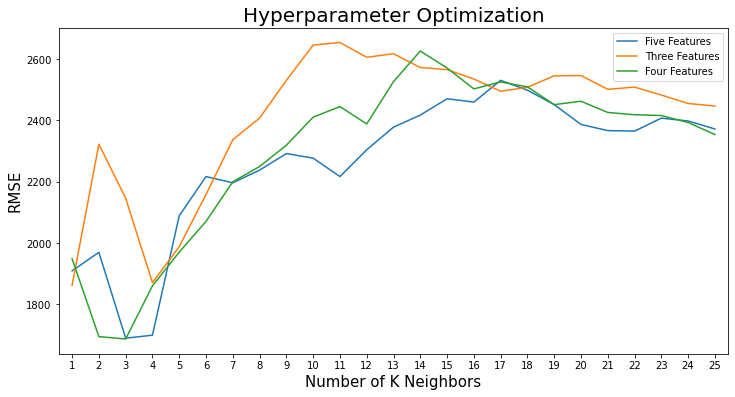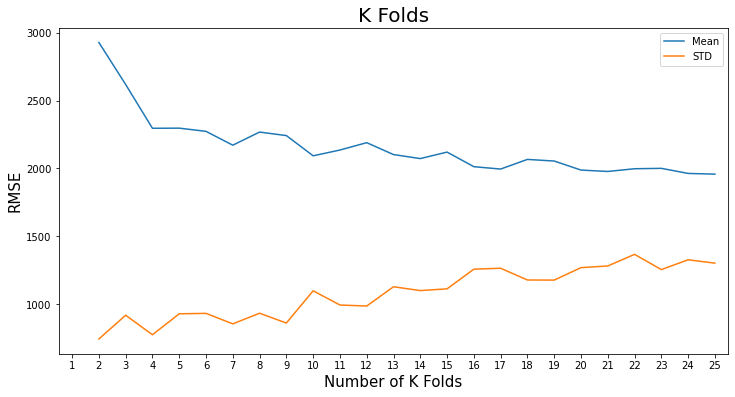# Predicting Car Prices¶

The goal of this project is to use K Nearest to predict the market price of cars based on a number of it's attributes. K nearest computes the elucidean distance to find similarity and average in order to predict unknown values.

The data set we will be working with contains information on various cars. For each car we have information about the technical aspects of the vehicle such as the motor's displacement, the weight of the car, the miles per gallon, how fast the car accelerates, and more. You can read more about the data set [here-https://archive.ics.uci.edu/ml/datasets/automobile].

Throughout the project I will be looking to achieve the lowest Square root mean squared error (RMSE). This will show that there is the smallest error between the price and the predicted price.

To start with we need to clean the data. Only numeric values will be useful for this project.

# Data Cleaning¶

In :
import pandas as pd
import numpy as np
pd.options.display.max_columns = None

# Rename columns
col_names = ["symboling", "normalized-losses", "make", "fuel-type", "aspiration", "num-of-doors", "body-style", "drive-wheels"
,"engine-location", "wheel-base", "length", "width", "height", "curb-weight", "engine-type", "num-of-cylinders", "engine-size"
,"fuel-system", "bore", "stroke", "compression-ratio", "horsepower", "peak-rpm", "city-mpg", "highway-mpg", "price"]
file = pd.read_csv("imports-85.data", names = col_names)

In :
file.head(5)

Out:
symboling normalized-losses make fuel-type aspiration num-of-doors body-style drive-wheels engine-location wheel-base length width height curb-weight engine-type num-of-cylinders engine-size fuel-system bore stroke compression-ratio horsepower peak-rpm city-mpg highway-mpg price
0 3 ? alfa-romero gas std two convertible rwd front 88.6 168.8 64.1 48.8 2548 dohc four 130 mpfi 3.47 2.68 9.0 111 5000 21 27 13495
1 3 ? alfa-romero gas std two convertible rwd front 88.6 168.8 64.1 48.8 2548 dohc four 130 mpfi 3.47 2.68 9.0 111 5000 21 27 16500
2 1 ? alfa-romero gas std two hatchback rwd front 94.5 171.2 65.5 52.4 2823 ohcv six 152 mpfi 2.68 3.47 9.0 154 5000 19 26 16500
3 2 164 audi gas std four sedan fwd front 99.8 176.6 66.2 54.3 2337 ohc four 109 mpfi 3.19 3.40 10.0 102 5500 24 30 13950
4 2 164 audi gas std four sedan 4wd front 99.4 176.6 66.4 54.3 2824 ohc five 136 mpfi 3.19 3.40 8.0 115 5500 18 22 17450
In :
# Replace '?'
file["num-of-doors"] = file["num-of-doors"].replace("four", 4).replace("two", 2).replace("?", np.nan)
file['num-of-cylinders'] = file['num-of-cylinders'].replace("four", 4).replace("six", 6).replace("five", 5).replace("eight", 8).replace("two", 2).replace("three", 3).replace("twelve", 12)
file = file.replace("?", np.nan)

In :
# Remove non numeric columns
numeric_cols = list(file.columns)
cols_to_drop = ["make", "fuel-type", "aspiration", "body-style", "drive-wheels", "engine-location", "engine-type", "fuel-system"]
for cols in cols_to_drop:
if cols in numeric_cols:
numeric_cols.remove(cols)


We also need to normalize the columns so that individual values do not overly affect the results. Below we will keep the same scale for values but ensure that all values are between 0 and 1. The only column that will remain the same is the price column as this is the target column for our analysis.

# Data Normalization¶

In :
normalized_cars = file[numeric_cols].copy()
# drop Na columns
normalized_cars.dropna(inplace=True)

# convert columns to numeric values
normalized_cars["price"] = normalized_cars["price"].astype(int)
normalized_cars["peak-rpm"] = normalized_cars["peak-rpm"].astype(int)
normalized_cars["horsepower"] = normalized_cars["horsepower"].astype(int)
normalized_cars["stroke"] = normalized_cars["stroke"].astype(float)
normalized_cars["bore"] = normalized_cars["bore"].astype(float)
normalized_cars["normalized-losses"] = normalized_cars["normalized-losses"].astype(int)

# Normalize columns between 0 and 1 except for target column price
saved_price = normalized_cars["price"]
normalized_cars = (normalized_cars - normalized_cars.min()) / (normalized_cars.max() - normalized_cars.min())
normalized_cars["price"] = saved_price

Out:
symboling normalized-losses num-of-doors wheel-base length width height curb-weight num-of-cylinders engine-size bore stroke compression-ratio horsepower peak-rpm city-mpg highway-mpg price
3 0.8 0.518325 1.0 0.455172 0.577236 0.517544 0.471154 0.329325 0.2 0.243655 0.464286 0.633333 0.18750 0.355263 0.551020 0.264706 0.333333 13950
4 0.8 0.518325 1.0 0.441379 0.577236 0.535088 0.471154 0.518231 0.4 0.380711 0.464286 0.633333 0.06250 0.440789 0.551020 0.088235 0.111111 17450
6 0.6 0.486911 1.0 0.662069 0.839024 0.973684 0.605769 0.525989 0.4 0.380711 0.464286 0.633333 0.09375 0.407895 0.551020 0.117647 0.194444 17710
8 0.6 0.486911 1.0 0.662069 0.839024 0.973684 0.625000 0.619860 0.4 0.355330 0.421429 0.633333 0.08125 0.605263 0.551020 0.058824 0.055556 23875
10 0.8 0.664921 0.0 0.503448 0.580488 0.394737 0.471154 0.351823 0.2 0.238579 0.685714 0.347619 0.11250 0.348684 0.673469 0.235294 0.305556 16430

Now that the dataset is ready lets begin. We will start by using a Univariate Model which only uses one attribute to train the data. For example, can we predict the price by finding the average price of the 5 cars with the most similar engine size.

# Univariate Model¶

In :
from sklearn.metrics import mean_squared_error
from sklearn.neighbors import KNeighborsRegressor

def knn_train_test(train_col, train_target, df):
np.random.seed(1)

# Randomize order of rows in data frame.
shuffled_index = np.random.permutation(df.index)
rand_df = df.reindex(shuffled_index)

# Assign test and train datasets
train_df = rand_df[:int((len(df) * 0.75))]
test_df = rand_df[int((len(df) * 0.75)):]

# Calculate Mean squared error
knn = KNeighborsRegressor()
knn.fit(train_df[[train_col]], train_df[train_target])
prediction = knn.predict(test_df[[train_col]])
mse = mean_squared_error(prediction, test_df[train_target])
return mse**(1/2)

rmses = {}

# list of columns
columns = list(normalized_cars.columns)
columns.remove('price')

# Use function on each column
for col in columns:
rmse = knn_train_test(col, "price", normalized_cars)
rmses[col] = rmse
rmses

Out:
{'symboling': 4728.749369759409,
'normalized-losses': 4417.589877750084,
'num-of-doors': 4695.416567249385,
'wheel-base': 2681.3032586785102,
'length': 2222.3324409277748,
'width': 2718.763254312519,
'height': 4193.069881244528,
'curb-weight': 2135.015488702599,
'num-of-cylinders': 4390.61656194207,
'engine-size': 2624.5118045076497,
'bore': 3903.751335574539,
'stroke': 4218.941348845703,
'compression-ratio': 6224.4305330688685,
'horsepower': 2211.671943801792,
'peak-rpm': 5212.425816066834,
'city-mpg': 2774.1447757822593,
'highway-mpg': 2249.4344375864794}
In :
# Visaulize RMSE's
import matplotlib.pyplot as plt

plt.figure(figsize=(12, 6))
plt.bar(list(rmses.keys()), rmses.values())
plt.xticks(rotation=90, size=15)
plt.ylabel("RMSE", size=15)
plt.xlabel("Car attributes", size=15)
plt.title("RMSE for each car attribute", size=20)

Out:
Text(0.5, 1.0, 'RMSE for each car attribute')We have a few attributes that are more accurate than the rest. The 5 attributes with the lowerst error in predciting the price are curb-weight, horsepower, length, highway-mpg and engine size. For each of these we looked at the 5 nearest neighbors. Let's see if we can get any more accurate by using the 1-10 closest neighbors.

In :
def knn_train_test(train_col, train_target, df, k_num):
np.random.seed(1)

# Randomize order of rows in data frame.
shuffled_index = np.random.permutation(df.index)
rand_df = df.reindex(shuffled_index)

# Assign test and train datasets
train_df = rand_df[:int((len(df) * 0.75))]
test_df = rand_df[int((len(df) * 0.75)):]

# Calculate Mean Squared Error
knn = KNeighborsRegressor(k_num)
knn.fit(train_df[[train_col]], train_df[train_target])
prediction = knn.predict(test_df[[train_col]])
mse = mean_squared_error(prediction, test_df[train_target])
return mse**(1/2)

# Test function on k neighbors 1-10
k_nums = list(range(1,11))

# Top 5 attributes
curb_weight = {}
for k in k_nums:
rmse = knn_train_test("curb-weight", "price", normalized_cars, k)
curb_weight[k] = rmse

horsepower = {}
for k in k_nums:
rmse = knn_train_test("horsepower", "price", normalized_cars, k)
horsepower[k] = rmse

length = {}
for k in k_nums:
rmse = knn_train_test("length", "price", normalized_cars, k)
length[k] = rmse

highway_mpg = {}
for k in k_nums:
rmse = knn_train_test("highway-mpg", "price", normalized_cars, k)
highway_mpg[k] = rmse

engine_size = {}
for k in k_nums:
rmse = knn_train_test("engine-size", "price", normalized_cars, k)
engine_size[k] = rmse

In :
# Visaulaize 1-10 K numbers
plt.figure(figsize=(12, 6))
plt.plot(list(engine_size.keys()), list(engine_size.values()), label='Engine Size')
plt.plot(list(highway_mpg.keys()), list(highway_mpg.values()), label='Highway-mpg')
plt.plot(list(length.keys()), list(length.values()), label='Length')
plt.plot(list(horsepower.keys()), list(horsepower.values()), label='Horsepower')
plt.plot(list(curb_weight.keys()), list(curb_weight.values()), label='Curb-weight')

plt.ylim(1500,5000)
plt.ylabel("RMSE (\$)", size=15)
plt.xlabel("K Number", size=15)
plt.xticks(range(1,11))
plt.title("RMSE for K Numbers 1-10", size=20)
plt.legend()

Out:
<matplotlib.legend.Legend at 0xadf2b38>Engine size is a bit of an outlier here. For the other 4 it looks like a k number of 7-8 brings the most accurate predictions whereas with engine size the most accurate K number is 4.

Let's now increase the number of attributes we use to make our predictions. For example, do we get less a smaller margin of error if we look at the cars with the most similar engine size, horsepower and highway-mpg rather than just one of these attributes.

# Multivariate Model¶

In :
def knn_train_test(train_cols, train_target, df):
np.random.seed(1)

# Randomize order of rows in data frame.
shuffled_index = np.random.permutation(df.index)
rand_df = df.reindex(shuffled_index)

# Assign test and train datasets
train_df = rand_df[:int((len(df) * 0.75))]
test_df = rand_df[int((len(df) * 0.75)):]
# Calculate Mean Squared Errors
knn = KNeighborsRegressor()
knn.fit(train_df[train_cols], train_df[train_target])
prediction = knn.predict(test_df[train_cols])
mse = mean_squared_error(prediction, test_df[train_target])
return mse**(1/2)

# 2,3,4,5 best attributes
two_best = ["curb-weight", "horsepower"]
three_best = ["curb-weight", "horsepower", "length"]
four_best = ["curb-weight", "horsepower", "length", "highway-mpg"]
five_best = ["curb-weight", "horsepower", "length", "highway-mpg", "engine-size"]

# Function on 2,3,4,5th best features
rmse_two_best = knn_train_test(two_best, 'price', normalized_cars)
rmse_three_best = knn_train_test(three_best, 'price', normalized_cars)
rmse_four_best = knn_train_test(four_best, 'price', normalized_cars)
rmse_five_best = knn_train_test(five_best, 'price', normalized_cars)

print("RMSE with 2 best features: {}".format(rmse_two_best))
print("RMSE with 3 best features: {}".format(rmse_three_best))
print("RMSE with 4 best features: {}".format(rmse_four_best))
print("RMSE with 5 best features: {}".format(rmse_five_best))

RMSE with 2 best features: 2114.83344237791
RMSE with 3 best features: 1987.7541040078372
RMSE with 4 best features: 1970.0850007042845
RMSE with 5 best features: 2088.677455712107


These numbers are coming down. Let's continue to look for the lowest RMSE by using hyperparameter optimization whichlooks for the optimal hyperparameter value (i.e nest number of k neighbors). We are going to loop through 1-25 K neighbors along with the 3 best multivariate lists of attributes we found above.

# Hyperparameter Optimization¶

In :
def knn_train_test(train_cols, train_target, df, k_num):
np.random.seed(1)

# Randomize order of rows in data frame.
shuffled_index = np.random.permutation(df.index)
rand_df = df.reindex(shuffled_index)

# Assign test and train datasets
train_df = rand_df[:int((len(df) * 0.75))]
test_df = rand_df[int((len(df) * 0.75)):]

# Calculate mean squared errors
knn = KNeighborsRegressor(k_num)
knn.fit(train_df[train_cols], train_df[train_target])
prediction = knn.predict(test_df[train_cols])
mse = mean_squared_error(prediction, test_df[train_target])
return mse**(1/2)

k_nums = list(range(1, 26))

with_top_five = {}
with_top_three = {}
with_top_four = {}

# Funtion on top 3 features
for k in k_nums:
result = knn_train_test(five_best, 'price', normalized_cars, k)
with_top_five[k] = result
for k in k_nums:
result = knn_train_test(three_best, 'price', normalized_cars, k)
with_top_three[k] = result
for k in k_nums:
result = knn_train_test(four_best, 'price', normalized_cars, k)
with_top_four[k] = result

print(with_top_five)
print("\n------------------------------------------------------------------------------------")
print(with_top_three)
print("\n------------------------------------------------------------------------------------")
print(with_top_four)

{1: 1908.7894986090007, 2: 1969.4308822601517, 3: 1689.1839145641372, 4: 1699.0605547625428, 5: 2088.677455712107, 6: 2216.58653050636, 7: 2196.2768958082734, 8: 2237.6074520288407, 9: 2291.708656180976, 10: 2276.801462139376, 11: 2216.5546668457564, 12: 2304.1030901039103, 13: 2377.847499850312, 14: 2417.2069982096855, 15: 2470.4970267809135, 16: 2459.711975643693, 17: 2530.581472647321, 18: 2498.490186808875, 19: 2451.4004451862365, 20: 2386.7422938233194, 21: 2366.631875259268, 22: 2364.885785390959, 23: 2407.2605812715133, 24: 2397.9911035271766, 25: 2371.8352317056097}

------------------------------------------------------------------------------------
{1: 1862.019622614112, 2: 2322.114474191572, 3: 2145.8102879021403, 4: 1870.5371383475656, 5: 1987.7541040078372, 6: 2158.100359691365, 7: 2336.875224699925, 8: 2407.477395078197, 9: 2530.6221487761745, 10: 2645.6187748804628, 11: 2654.205292767879, 12: 2605.759850159141, 13: 2617.5227623160417, 14: 2572.4166082951365, 15: 2565.5870205254955, 16: 2535.0711033852185, 17: 2495.0474085371106, 18: 2508.04797495501, 19: 2545.3008172875684, 20: 2546.2789382008013, 21: 2501.2579576808303, 22: 2508.576377445437, 23: 2482.7204069323047, 24: 2455.0596943144924, 25: 2446.7601700452788}

------------------------------------------------------------------------------------
{1: 1948.5735872683895, 2: 1694.3284049292215, 3: 1686.665338438212, 4: 1860.036953177947, 5: 1970.0850007042845, 6: 2070.5084265572177, 7: 2199.5210471224627, 8: 2249.4048259692495, 9: 2318.8411322479465, 10: 2410.4803709530597, 11: 2444.998685628946, 12: 2388.432660181614, 13: 2526.4479851945284, 14: 2626.2851186347307, 15: 2570.8897807525273, 16: 2502.68424556671, 17: 2525.2735766179285, 18: 2509.3489598836463, 19: 2451.3118809331213, 20: 2462.4268248391663, 21: 2425.8419942264136, 22: 2418.3242936283154, 23: 2415.7340875416676, 24: 2393.064093354901, 25: 2353.697030129409}

In :
# Visualize Hyperparameter Optimization
plt.figure(figsize=(12,6))

plt.plot(list(with_top_five.keys()), list(with_top_five.values()), label = "Five Features")
plt.plot(list(with_top_three.keys()), list(with_top_three.values()), label = "Three Features")
plt.plot(list(with_top_four.keys()), list(with_top_four.values()), label = "Four Features")
plt.legend()
plt.xticks(range(1, 26))
plt.xlim(0.5,25.5)
plt.ylabel("RMSE", size=15)
plt.xlabel("Number of K Neighbors", size=15)
plt.title("Hyperparameter Optimization", size=20)

Out:
Text(0.5, 1.0, 'Hyperparameter Optimization')The lowest RMSE's are found in the lower k neighbors (from 2-4). The singular lowest score came from using the attributes "curb-weight", "horsepower", "length", "highway-mpg", "engine-size" together along with a k number of 3. Throughout this project we have used the 75-25 train and test method in which you use 75% of the dataset to train the data and then the other 25% to test it. Let's finish by using a different technique k-folds. k-folds involves the following:

• splitting the full dataset into k equal length partitions.
• selecting k-1 partitions as the training set and
• selecting the remaining partition as the test set
• training the model on the training set.
• using the trained model to predict labels on the test fold.
• computing the test fold's error metric.
• repeating all of the above steps k-1 times, until each partition has been used as the test set for an iteration.
• calculating the mean of the k error values.

Below we are going to loop through 1-25 k folds to see which gives the lowest RMSE.

In :
from sklearn.model_selection import cross_val_score, KFold

# K fold function
def knn_train_test_kfold(train_cols, train_target, df, folds):
rmses = []
kf = KFold(folds, shuffle=True, random_state=1)
model = KNeighborsRegressor(3)
mses = cross_val_score(model, df[train_cols], df[train_target],
scoring="neg_mean_squared_error", cv=kf)
mses = list(mses)
for v in mses:
v = abs(v)
rmse = v**(1/2)
rmses.append(rmse)
return rmses, np.mean(rmses), np.std(rmses)

k_folds = list(range(2,26))

k_folds_means = {}
k_folds_std = {}

# Loop through number of k folds
for n in k_folds:
s = knn_train_test_kfold(five_best, "price", normalized_cars, n)
k_folds_means[n] = s
k_folds_std[n] = s

print(k_folds_means)
print("\n------------------------------------------------------------------")
print(k_folds_std)

{2: 2927.3460555374886, 3: 2618.0553343482184, 4: 2295.8348274216028, 5: 2296.8260632154092, 6: 2273.234462030219, 7: 2171.456088833527, 8: 2267.954964088096, 9: 2242.140196500448, 10: 2093.263396540541, 11: 2135.7549883282413, 12: 2189.9510900938294, 13: 2102.414340454889, 14: 2072.8020339088766, 15: 2120.654092397812, 16: 2013.2140599786032, 17: 1995.7138397821452, 18: 2066.769798812635, 19: 2054.7957734427146, 20: 1988.3368466236711, 21: 1978.008211081012, 22: 1998.0681329217625, 23: 2001.0309155202178, 24: 1963.5786094089592, 25: 1958.4501941005008}

------------------------------------------------------------------
{2: 745.3664165705284, 3: 920.0574269069089, 4: 776.4880430314498, 5: 930.6223722413541, 6: 933.8154774994462, 7: 856.6044312913886, 8: 934.8659556664095, 9: 862.7296727202488, 10: 1099.8567127719336, 11: 995.2862605124948, 12: 987.744682988567, 13: 1129.6650747844253, 14: 1101.5545453651685, 15: 1114.2675399403688, 16: 1258.9184665180949, 17: 1265.8878755038347, 18: 1179.319161838164, 19: 1178.6384549055665, 20: 1270.2883183902757, 21: 1282.5755421191684, 22: 1367.9638554287683, 23: 1256.0214344967953, 24: 1328.09119919684, 25: 1303.4944182840031}

In :
# Visualize K folds
plt.figure(figsize=(12,6))

plt.plot(list(k_folds_means.keys()), list(k_folds_means.values()), label='Mean')
plt.plot(list(k_folds_std.keys()), list(k_folds_std.values()), label='STD')
plt.legend()
plt.xticks(range(1, 26))
plt.xlim(0.5,25.5)
plt.ylabel("RMSE", size=15)
plt.xlabel("Number of K Folds", size=15)
plt.title("K Folds", size=20)

Out:
Text(0.5, 1.0, 'K Folds')In this graph we have the mean RSME along with the standard deviation. A low mean RMSE is good but not if it comes with high variance. Likewise, a low variance is desriable but only if it comes with a low mean. There is usually a trade off between the two. In the graph above I would recommend 7 k folds as the variance is still low and the mean has begun to drop towards it's lowest point.

# Conclusion¶

Throghout this project the closest we got in predicting the price was to use the attributes "curb-weight", "horsepower", "length", "highway-mpg", "engine-size" together along with a k number of 3. This predicted the market price of each car to an RMSE of 1686.66.